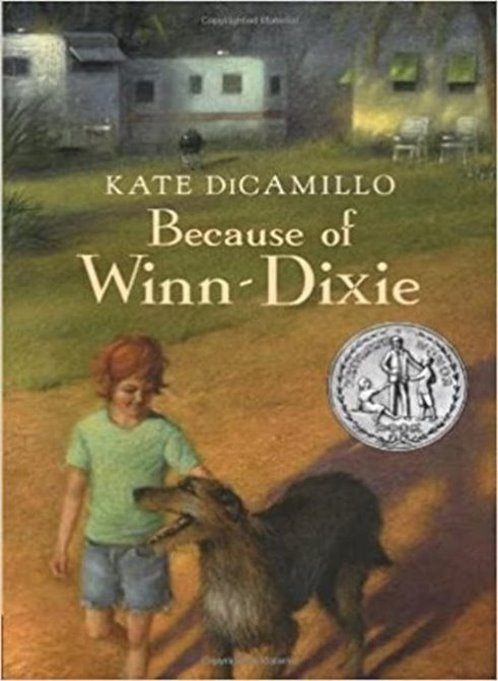## 3rd Grade- Because of Winn-DixieWe have started our Character Study Unit. We will be reading Because of Winn-Dixie and focusing on the main character, Opal. I will post the power point presentations to this website. Remember to listen to the chapter via Class Dojo and then answer the questions from the power point. I hope you enjoy Because of Winn-Dixie as much as I do!

Winn DIxie Chapter 1.pdf

Chapter 2 Power Point.pdf

Chapter 3 Powerpoint.pdf

Chapter 4 Power Point.pdf

Chapter 5 Power Point.pdf

Chapter 6 Power Point.pdf

Chapter 7 Power Point.pdf

Chapter 8 Power Point.pdf

Chapter 9 Power Point.pdf

Chapter 10 Power Point.pdf

Chapter 11 Power Point.pdf

Chapter 12 Power Point.pdf

Chapter 13 Power Point.pdf

Chapter 14 Power Point.pdf

Chapter 15 Power Point.pdf

Chapter 16 Power Point.pdf

Chapter 17 Power Point.pdf

Chapter 18 Power Point.pdf

Chapter 19 Power Point.pdf

Chapter 20 Power Point.pdf

Chapter 21 Power Point.pdf

Chapter 22 Power Point.pdf

Chapter 23 Power Point.

Chapter 24 Power Point.pdf

Chapter 25 Power Point.pdf

Chapter 26 Power Point.pdf

Winn Dixie Final Writing Assignment.pdf